### Montessori Material Spotlight: The Binomial and Trinomial Cubes

by

One of the first things you notice when entering a Montessori classroom is the abundance of beautifully made learning materials, neatly arranged on spacious shelves. The Montessori materials introduce children to abstract concepts and critical thinking skills using concrete materials. Two of Montessori's sensorial materials, the binomial and trinomial cubes, visually introduce the child to the concept of cubes of binomials and trinomials, while providing a fun challenge.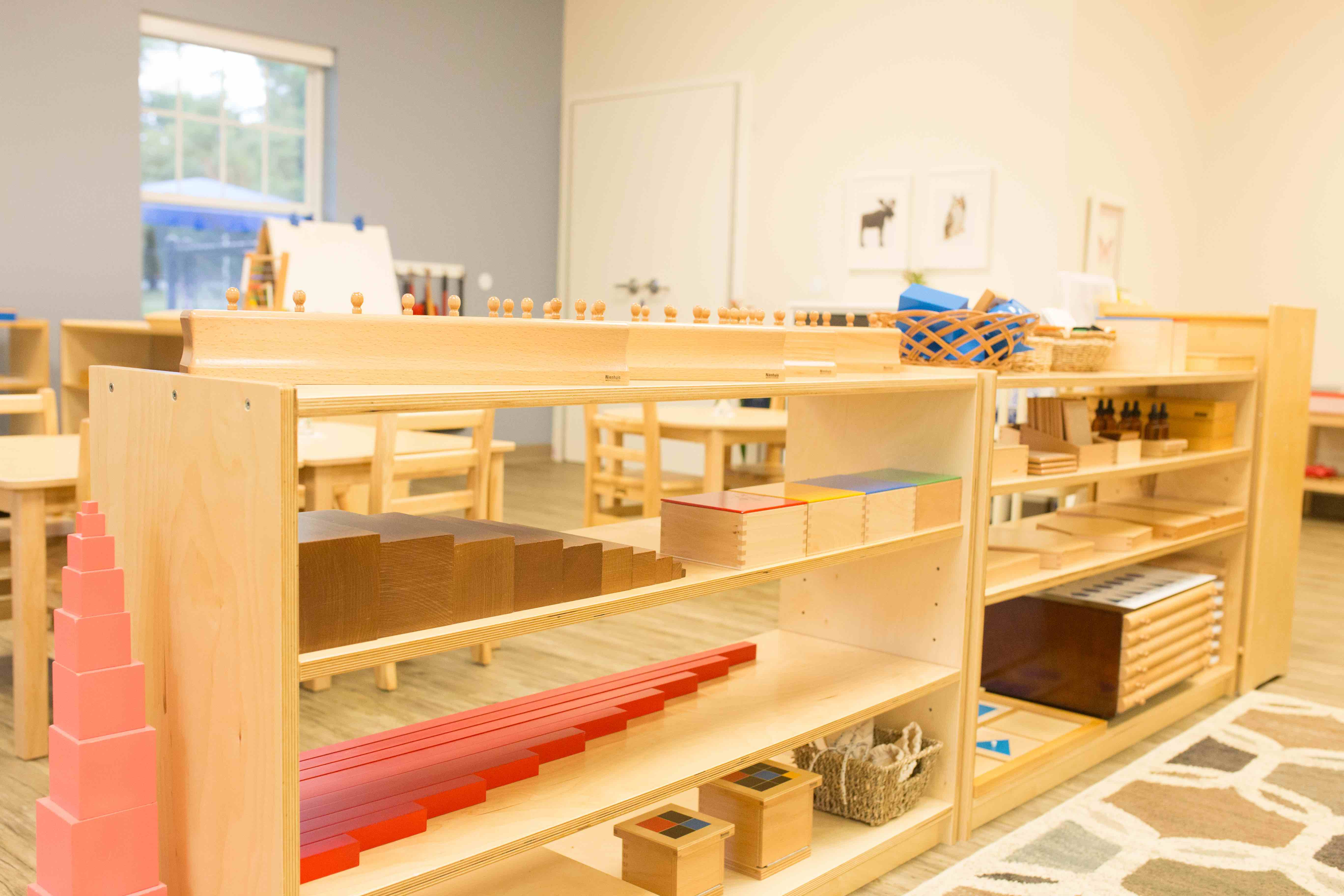What are the Binomial and Trinomial Cubes?

The binomial cube, when first introduced to the child, is presented as a challenging, three-dimensional puzzle. The cube is made up of a number of colored blocks, which fit together in a specific way. Assembling it uses a child's fine-motor skills and requires the ability to discriminate between the blocks based on multiple characteristics. Unlike Montessori's iconic pink tower, for example, the binomial cube does not isolate only one quality. Some blocks have one color, others have two. Some blocks are cubes, while others are rectangular prisms. While the pink tower blocks vary only in size, the binomial cube’s blocks vary in color, size, and shape! This makes the binomial cube a more complex sensorial material, and it requires organized thinking to master. The binomial cube's big brother, the trinomial cube, is a child's more complex next step.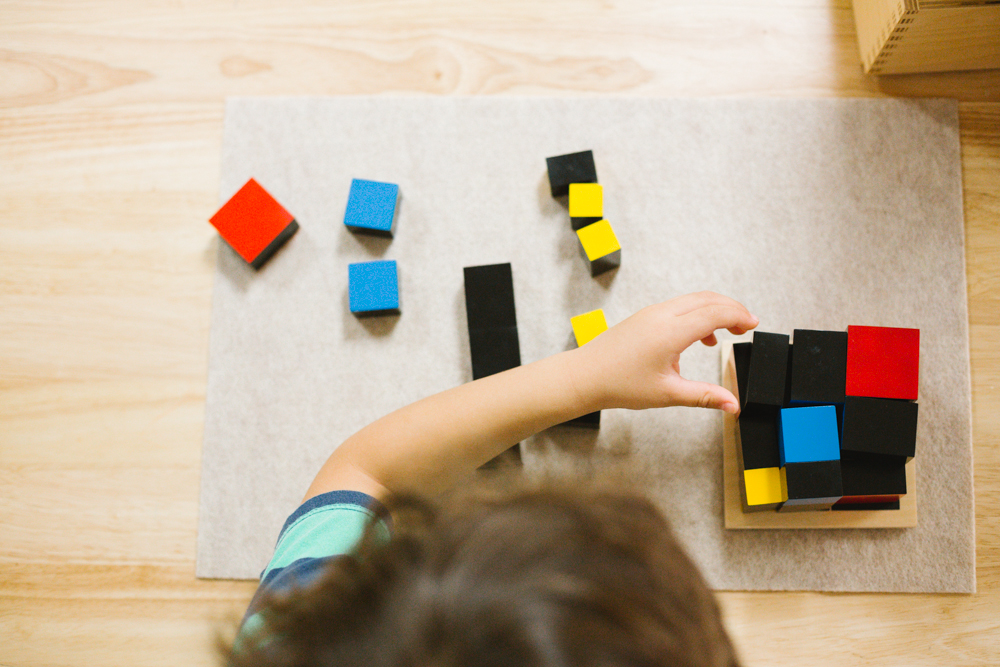Like other sensorial materials, the binomial and trinomial cubes are self correcting: when properly assembled, the blocks form a cube that fits perfectly inside of its wooden box! Even if the cube is built outside of its box, visual cues alert the child to any errors they might have made.

Children return to the cubes time after time, manipulating them with a focused sense of purpose. After a child has mastered building the binomial or trinomial cube inside of the box, he may then try building it outside of the box, or building each layer separately in order to observe similarities in patterns. Over time, the child’s familiarity with the cube’s physical aspects will lead to an internalized understanding of the abstract concepts the cube represents.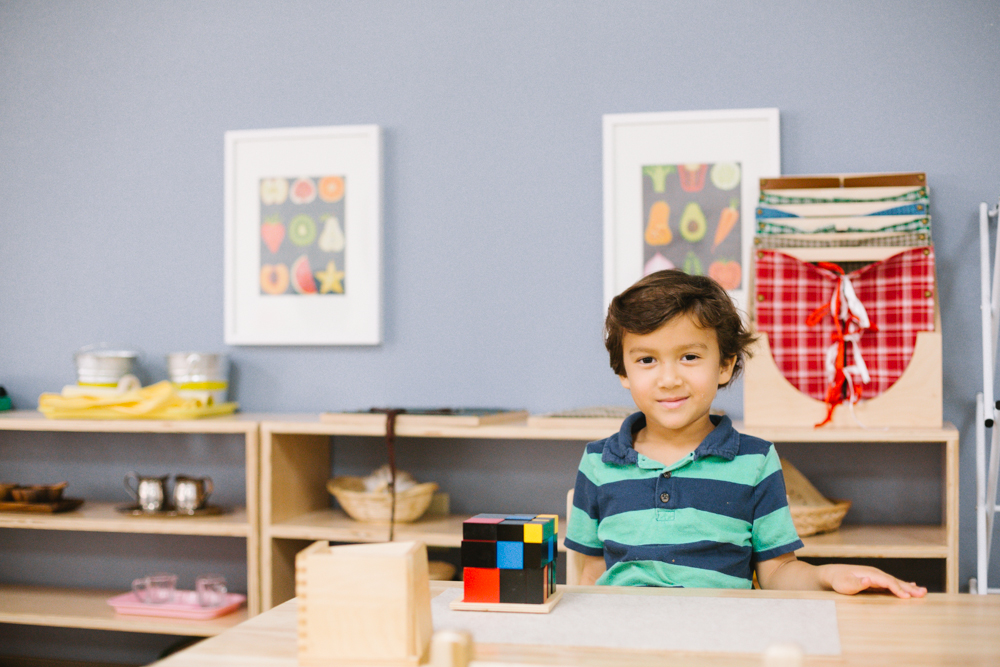Abstract Concepts Through Concrete Challenges

The cool thing about the binomial and trinomial cubes is that they prepare your child to learn advanced mathematical concepts! To the young child, the binomial cube is an entertaining three-dimensional puzzle, but more fundamentally, it is a concrete representation of the cube of a simple binomial equation: (a+b)3. The eight wooden blocks represent the expansion of this equation. The top of the box is painted to show what the assembled cube looks like from the top. Each block represents a component of the equation a3+ 3a2b + 3b2a + b3: a red cube representing a3, a smaller blue cube representing b3, three red and black cubes representing a2b, and three blue and black cubes representing b2a.

As with all Montessori sensorial materials, the cube has both a direct and an indirect purpose. The direct purpose of the binomial and trinomial cubes is for the child to practice the steps to properly disassemble and build the cube, while refining dexterity and visual acuity. Indirectly, the binomial cube prepares the child for higher-level math through concrete and visual exposure to the algebraic concept of a binomial cube. The indirect purpose in the Children’s House becomes the direct purpose when the material is re-introduced in the elementary classrooms. Elementary-aged children use the cube to develop an understanding of the expanded equation (a+b)<sup>3 </sup>= a<sup>3 </sup>+ 3a<sup>2</sup>b + 3b<sup>2</sup>a + b<sup>3</sup>. They then consider the trinomial equation: (a+b)= a+ 3a2b + 3b2a + b3. They then consider the trinomial equation: (a+b+c)= a+ 3a2b + 3a2b + 6abc + 3c2a + b+ 3b2c + 3c2b + c3. In traditional schools, these concepts are not usually introduced until junior high.

By the time a child reaches upper elementary, the advanced cubing materials are introduced, and the child learns to think about progressively more complicated relationships between cubes of different numbers. Finally, the students circle back to their old friends, the binomial and trinomial cubes, and the more abstract algebraic equations associated with them.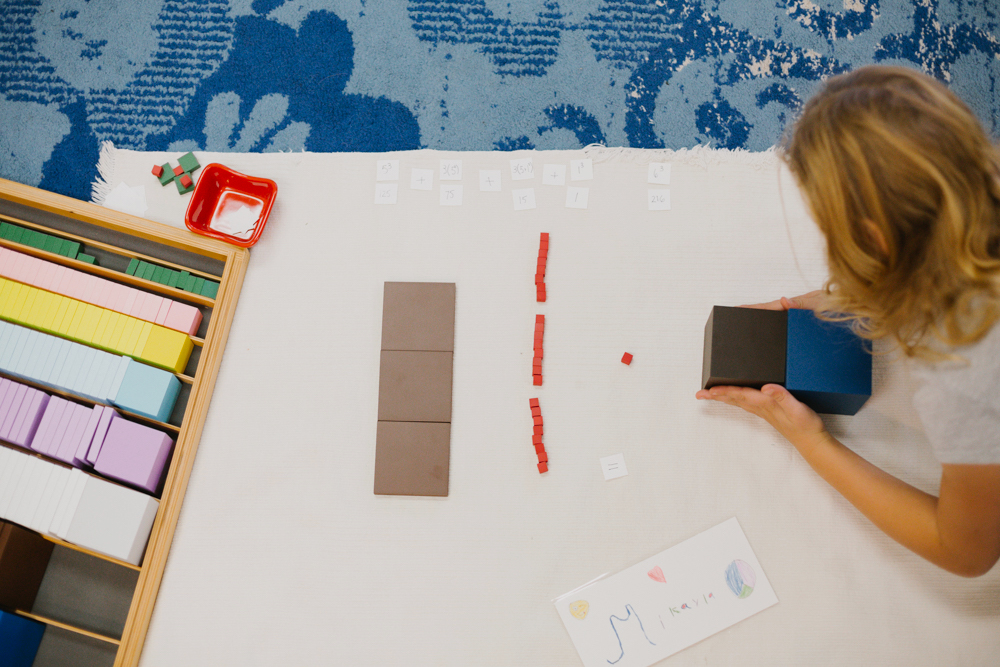The indirect purpose of the binomial and trinomial cubes in the Children’s House becomes the direct purpose when the material is re-introduced in the elementary classrooms. Elementary-aged children use the cube to develop an understanding of the expanded equation (a+b)= a+ 3a2b + 3b2a + b3.

They then consider the trinomial equation: (a+b+c)= a+ 3a2b + 3a2b + 6abc + 3c2a + b+ 3b2c + 3c2b + c3. In traditional schools, these concepts are not usually introduced until junior high.

Abstract understanding of concepts of numbers and formulas develops from a child’s interactions and experiences with real-life objects. By working with the sensorial materials, children learn to generalize and abstract from observed physical relationships, gradually progressing to greater levels of conceptual complexity. They build a solid, grounded foundation upon which they will build new knowledge. This mental process of forming concepts from abstractions is one that children will use continuously, and in every domain, for the rest of their lives.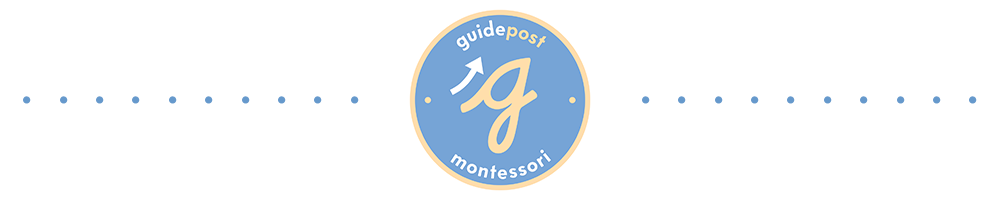Guidepost Montessori is a a global network of schools serving 3,500+ families with early education programs, including Emergency Care for Essential Workers (ECEW) during the COVID-19 crisis. Looking for more free content? Download Guidepost Parent from the App Store for personalized Montessori inspiration and activities.